National Institute of Standards and Technology
 Computational Chemistry Comparison and Benchmark DataBase Release 22 May 2022 NIST Standard Reference Database 101
 I Introduction II Experimental data III Calculated data IV Data comparisons V Cost comparisons VI Input and output files VII Tutorials and Units VIII Links to other sites IX Feedback X Older CCCBDB versions XII Geometries XIII Vibrations XIV Reaction data XV Entropy data XVI Bibliographic data XVII Ion data XVIII Bad calculations XIX Index of properties XX H-bond dimers XXI Oddities

NIST policy on privacy, security, and accessibility.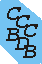1. 2. 3. 4. A. Geometry 1. Cartesians, bond lengths and angles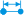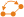a. Bond lengths of isoelectronic diatomics b. Bond angles of isoelectronic triatomics c. Compare specific calculated bonds or angles 2. Rotation and Moments of Inertia a. Rotational Constants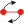b. Products of moments of inertiac. Calculated moments of inertiad. Calculated inertial defects e. Calculated moments of inertia and eigenvectors f. Calculated second moments 3. Point Group 4. Bond orders from AIM analysis 5. Average value of r squared ( ) 6. Number of conformers with calculated results 7. Just show me a calculated geometry 8. z-matrix B. Vibrations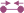1. Vibrational frequencies 2. Vibrational zero-point energies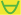3. Vibrational scaling factors.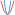a. List vibrational scaling factors. b. List vibrational scaling factors and their uncertainties. c. Calculate a vibrational scaling factor for a given set of molecules. d. Why scale vibrational frequencies? 4. Lookup molecules by their calculated vibrational frequencies. 5. Compare reduced masses for a given vibrational mode. 6. Animated vibrational modes.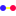7. Bad Vibrational Frequency calculations a. By comparing HF and MP2 calculations b. By comparing with experiment c. By anharmonic constants 8. Anharmonic Vibrational Frequency calculations a. Compare triatomic anharmonic vibrational constants C. Entropies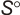1. Entropies and Integrated Heat Capacities at any temperature using calculated data. D. Energetics 1. Energies in hartrees a. Energies (equilibrium, no zero-point energy) b. Energies (equilibrium, no zero-point energy) with heat correction to 298.15K 2. Orbital Energies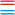a. HOMO-LUMO gap b. HOMO energy c. All Orbital Energies 3. Barriers to Internal Rotation and Inversion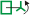a. Single species b. Compare two or more species c. Single species with dipole moment displayed 4. Nuclear repulsion Energies 5. Correlation Energies a. Partial Correlation Energies 6. Ionization Energies a. Electron Affinities b. Vertical Ionization Energies 7. Conformation Energies a. Minimum Energy conformation 8. Basis Set Extrapolation 9. Excited Electronic States 10. Singlet-Triplet Gap 11. Proton Affinities E. Electrostatics 1. Atomic charges a. Mulliken charges b. chelpg charges c. AIM charges d. Atom pair charge differences e. ESP charges 2. Dipole moments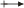a. Dipole Moment angles 3. Quadrupole moments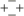4. Polarizabilities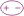5. Spin 6. Spin Density F. Information about calculations 1. List showing how many calculations are done by method/basis set. a. List showing how many calculations are done by molecule. 2. Calculations done for a given species. 3. Number of basis functions used in a given calculation 4. Calculated Diagnostics 5. Calculated Electronic State Symmetries G. Compare calculations Bond lengthsa. Bond lengths for one type of bond b. Selected bonds for several molecules c. Bond lengths of isoelectronic diatomics d. Bond angles of isoelectronic triatomics Compare calculated vibrational intensitiesCompare energy difference of a single point calculation Compare calculated transition state energies Compare dipoles calculated from atom chargesCalculated properties for several species Energy in hartrees Compare vibrational frequencies for two calculationsList of molecules sorted by size, as determined by DFT grid fineness comparisons a. Energy differences. b. Bond length differences. c. Point Group differences. Core correlation comparisons a. Energy differences. b. Bond length differences. Polarizability comparison Conformation comparison